# NCERT Solutions for Class 6 Maths Chapter 2 – Whole Numbers

Class 6 Maths NCERT Solutions Chapter 2 – Whole Numbers comprises of the 3 Exercises,

NCERT Solutions for Class 6 Maths Chapter 2 Exercise 2.1 – Video Explanation

NCERT Solutions for Class 6 Maths Chapter 2 Exercise 2.2 – Video Explanation

NCERT Solutions for Class 6 Maths Chapter 2 Exercise 2.3 – Video Explanation

This Chapter contains the Exercises relating to the following topics, which are discussed in Chapter 2 – Whole Numbers Class 6 NCERT book  : –

• Exercise 2.1 Introduction
• Exercise 2.2 Whole Numbers
• Exercise 2.3 The Number Line
• Exercise 2.4 Properties of Whole Numbers
• Exercise 2.5 Patterns in Whole Numbers

### NCERT Solutions for Class 6 Maths Chapter 2 Exercise 2.1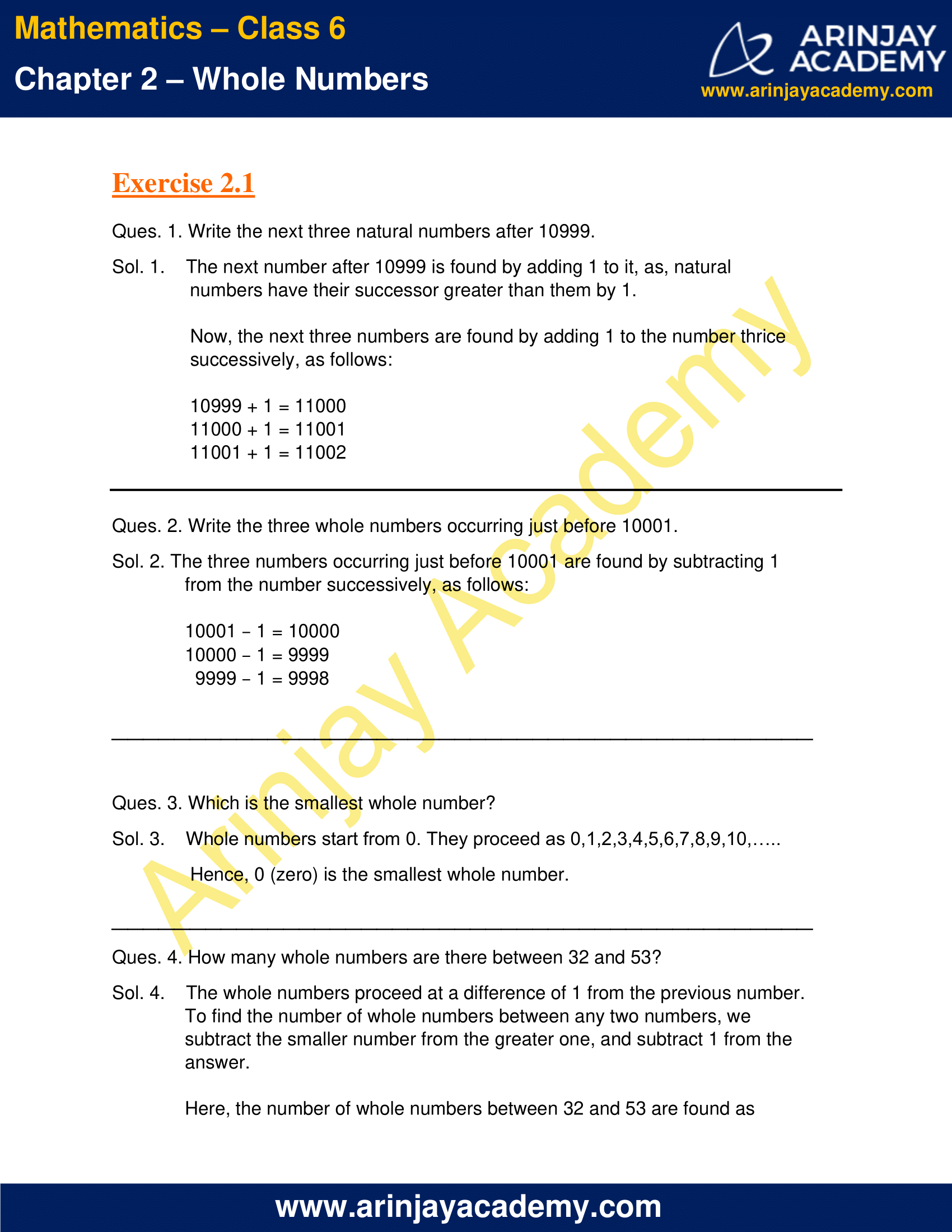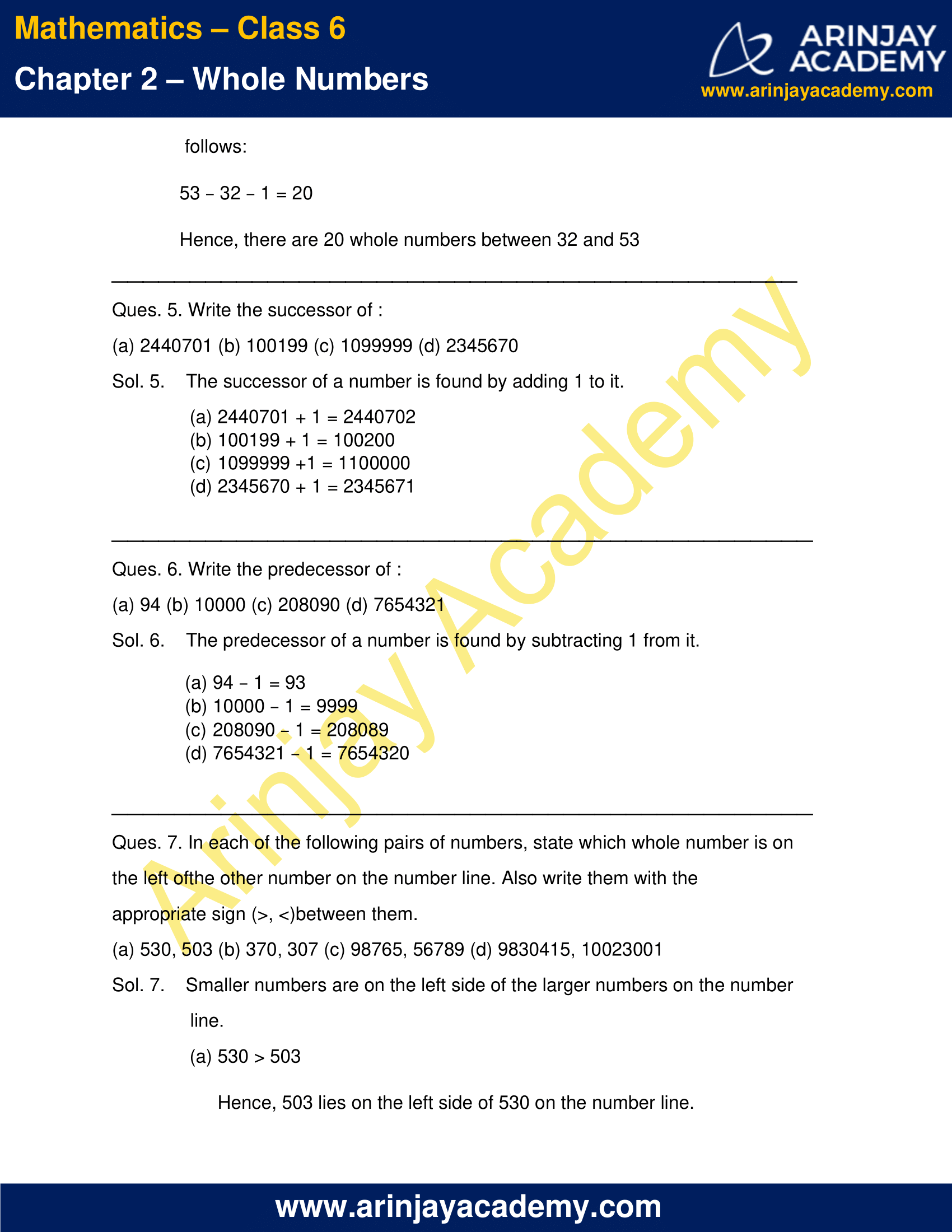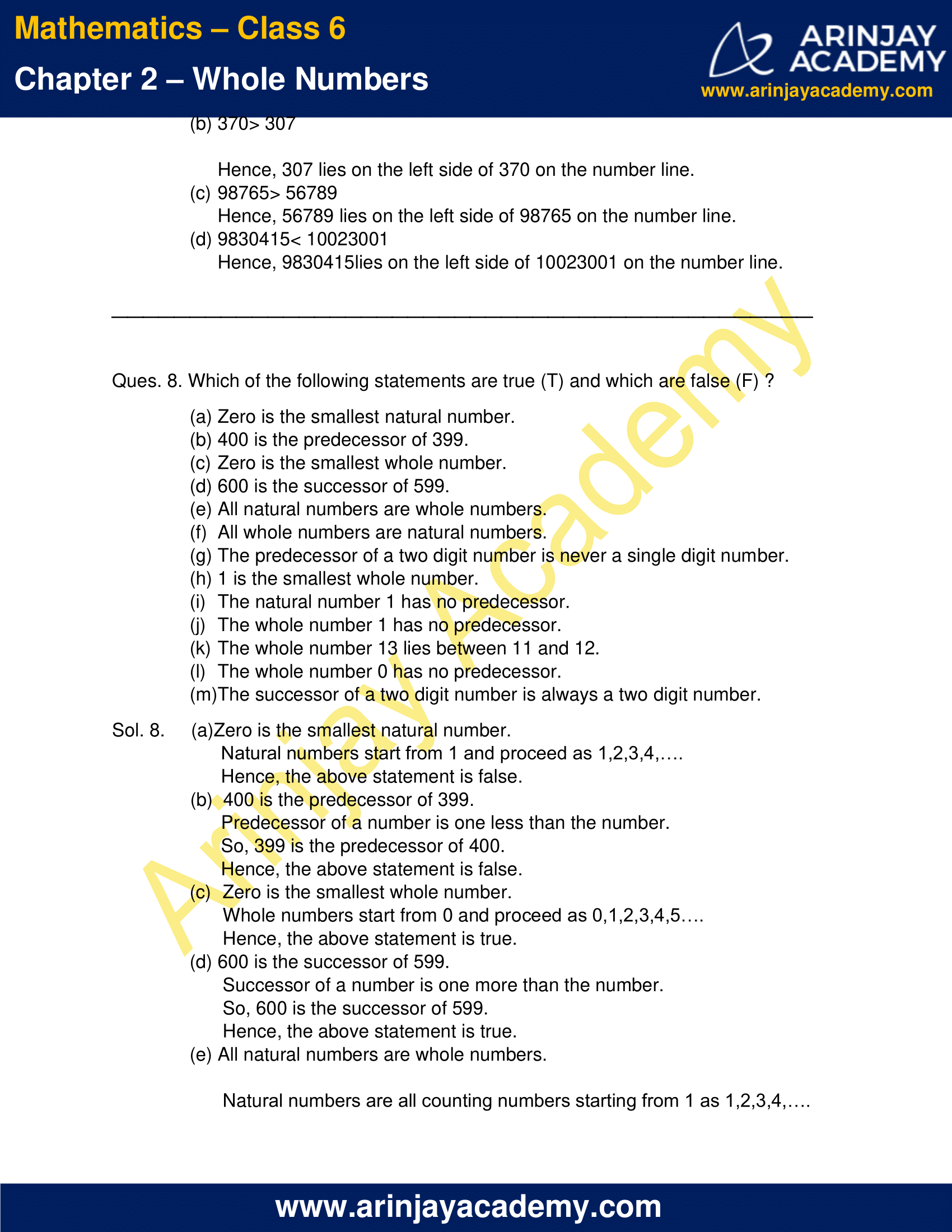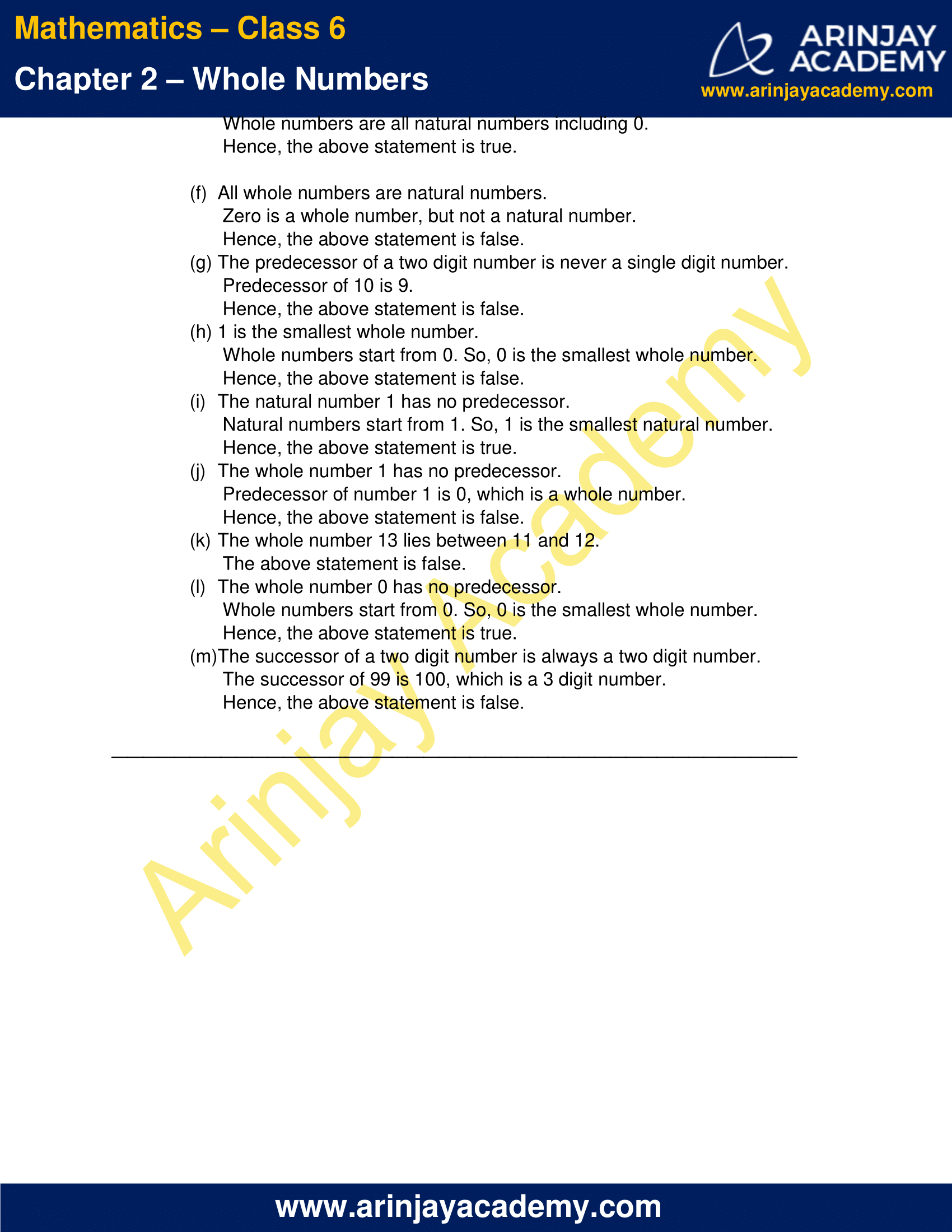### Class 6 Maths NCERT Solutions Chapter 2 – Exercise 2.2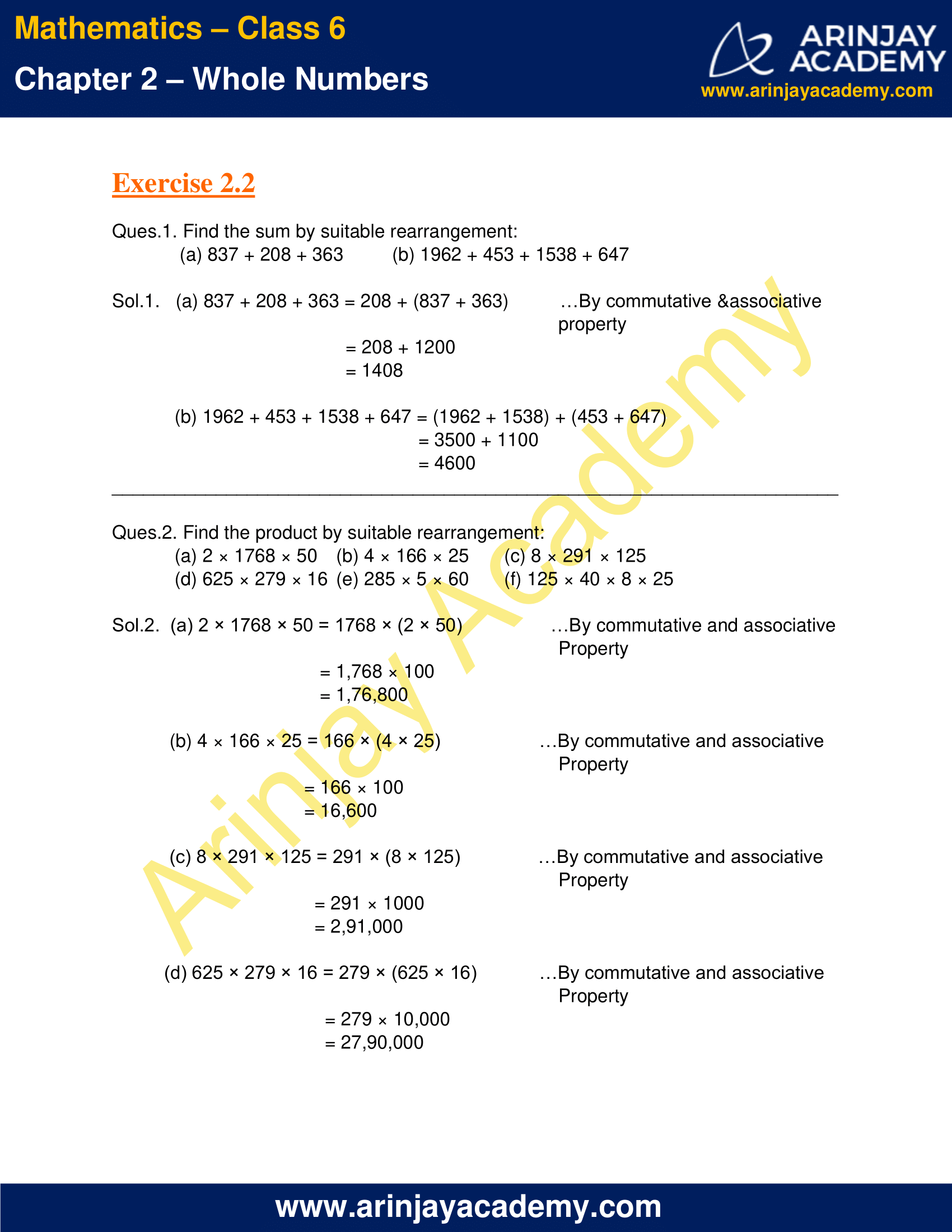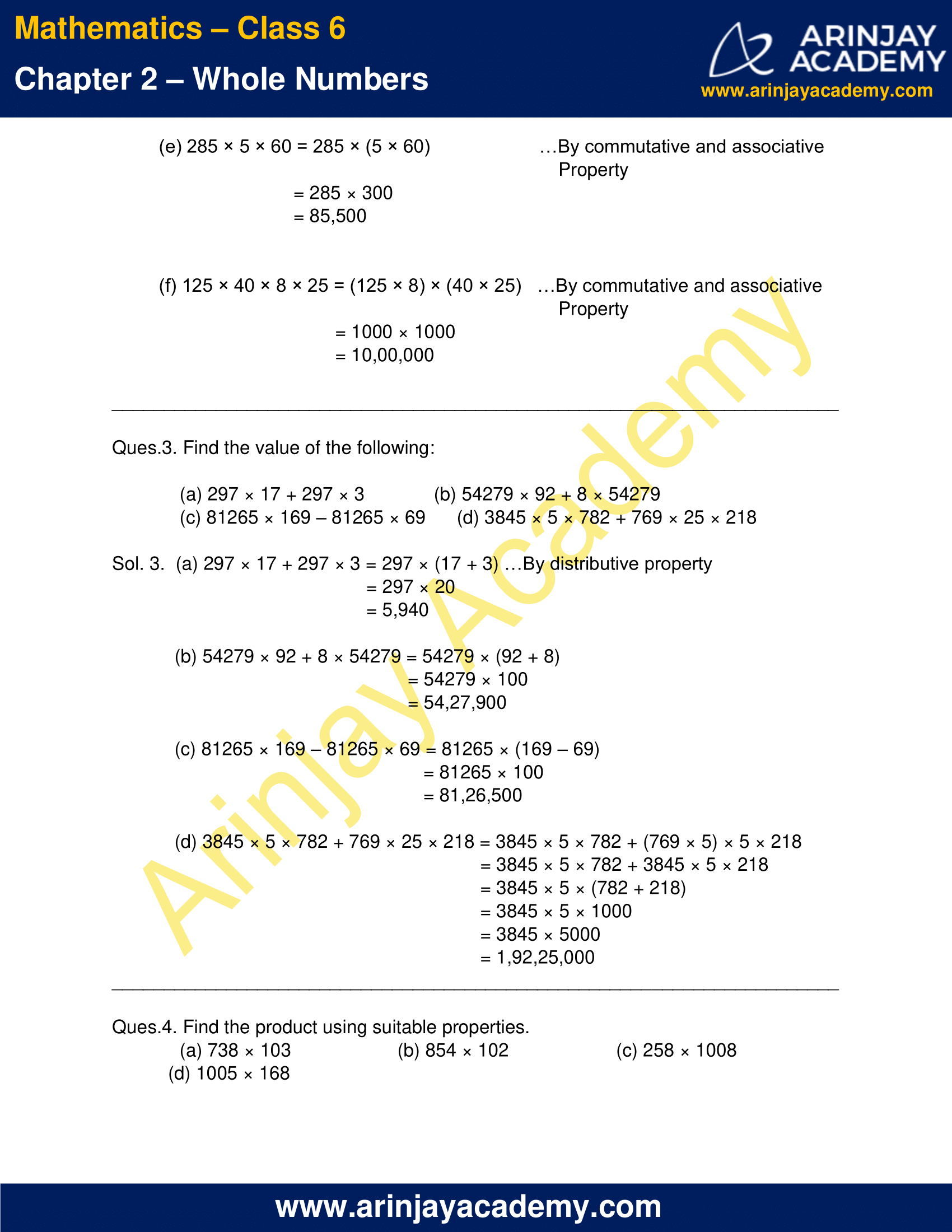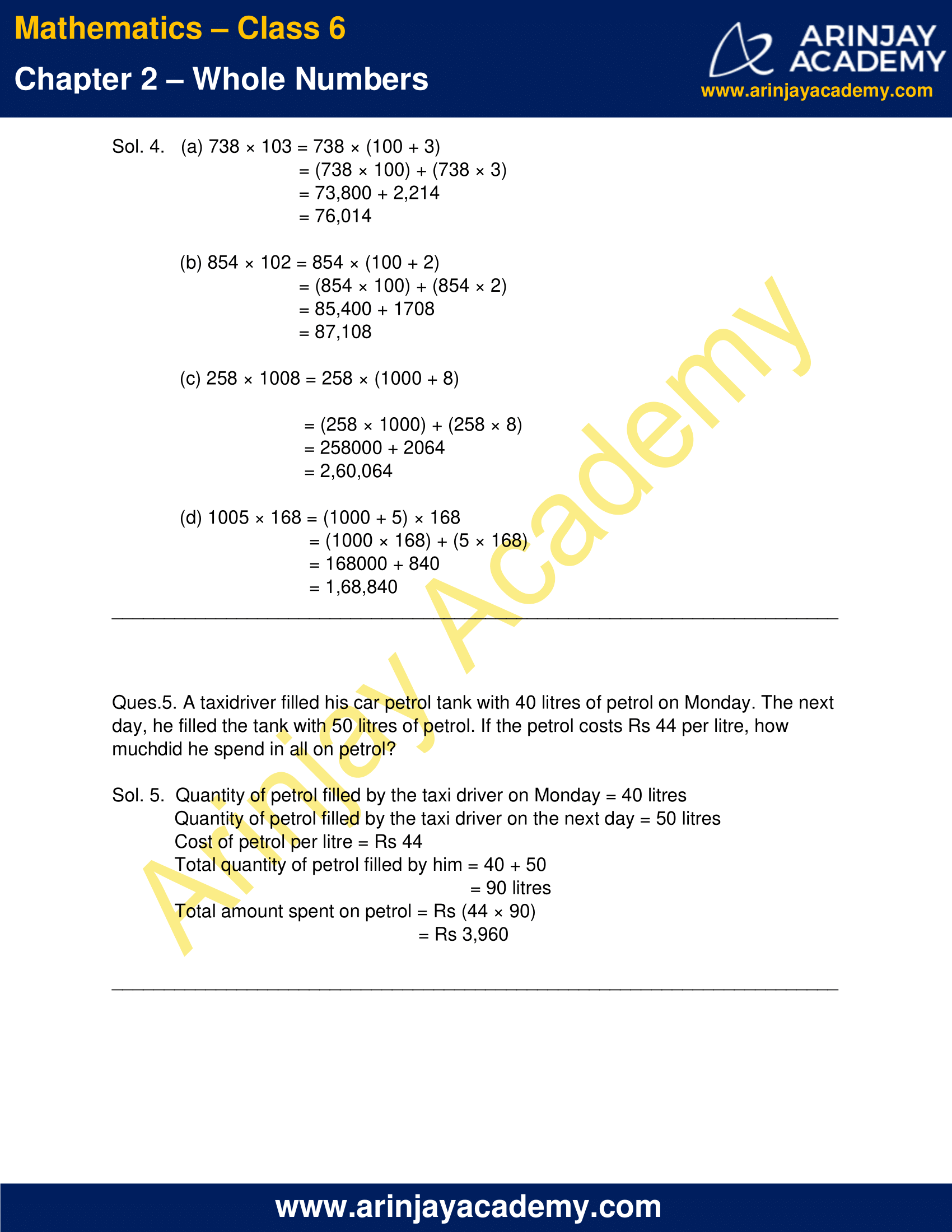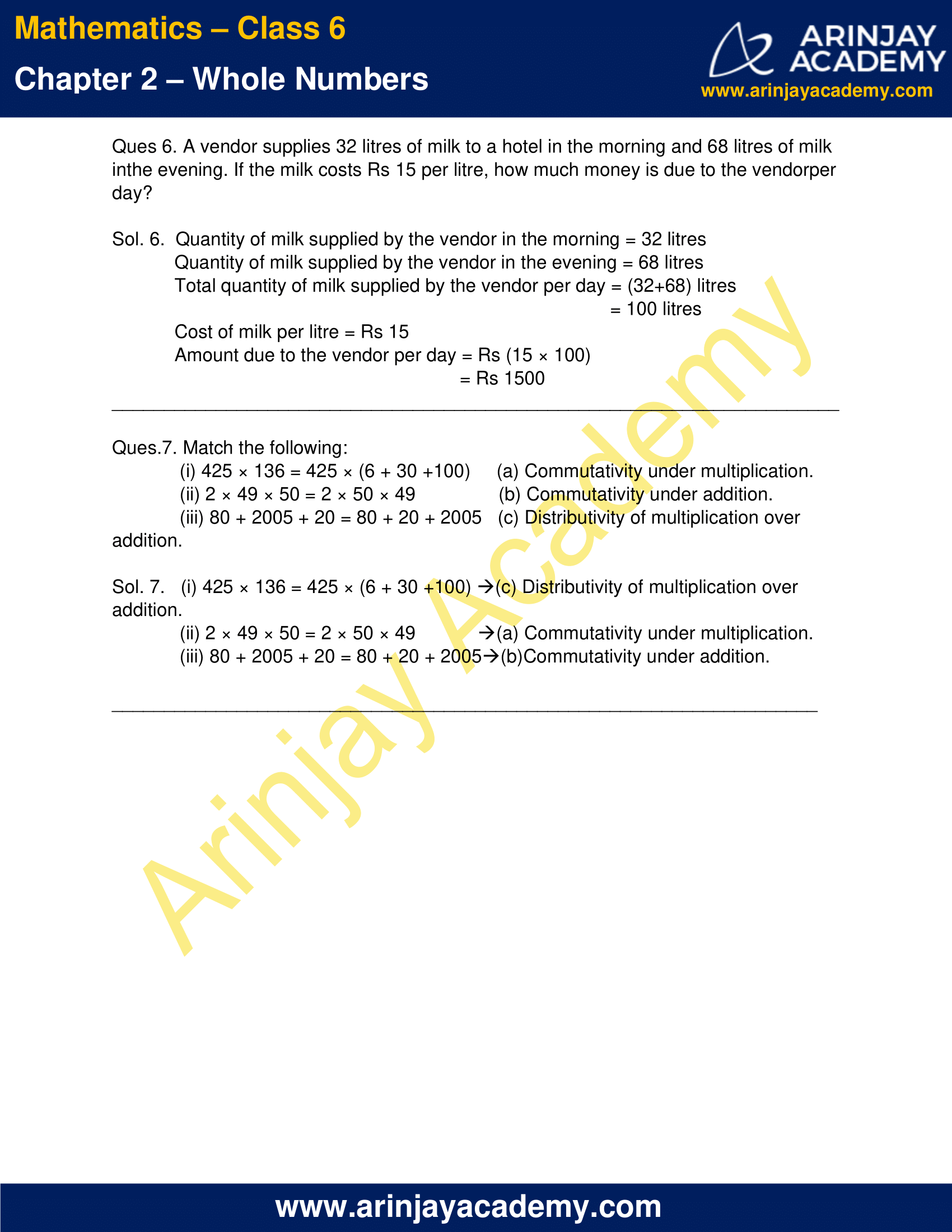### Class 6 Maths NCERT Solutions Chapter 2 – Exercise 2.3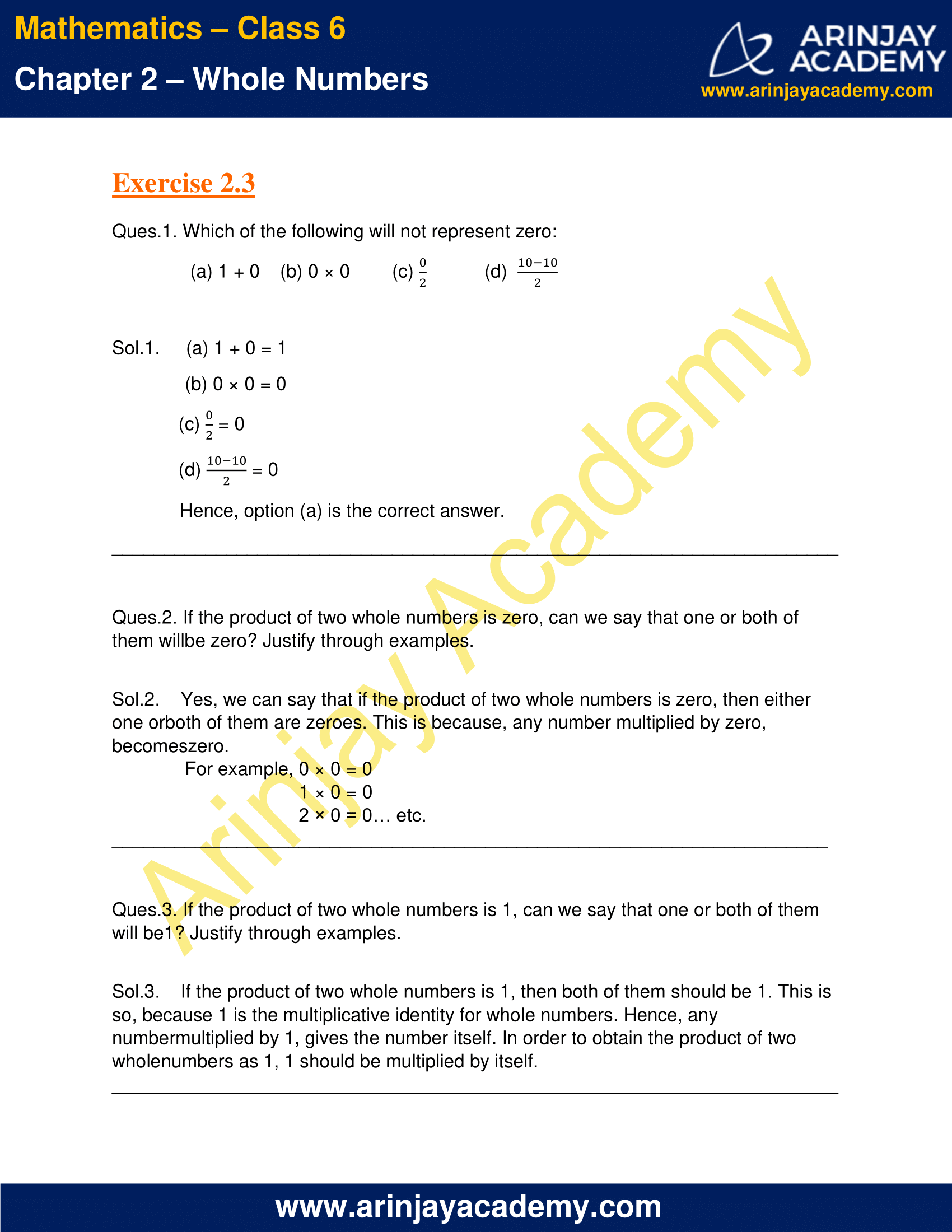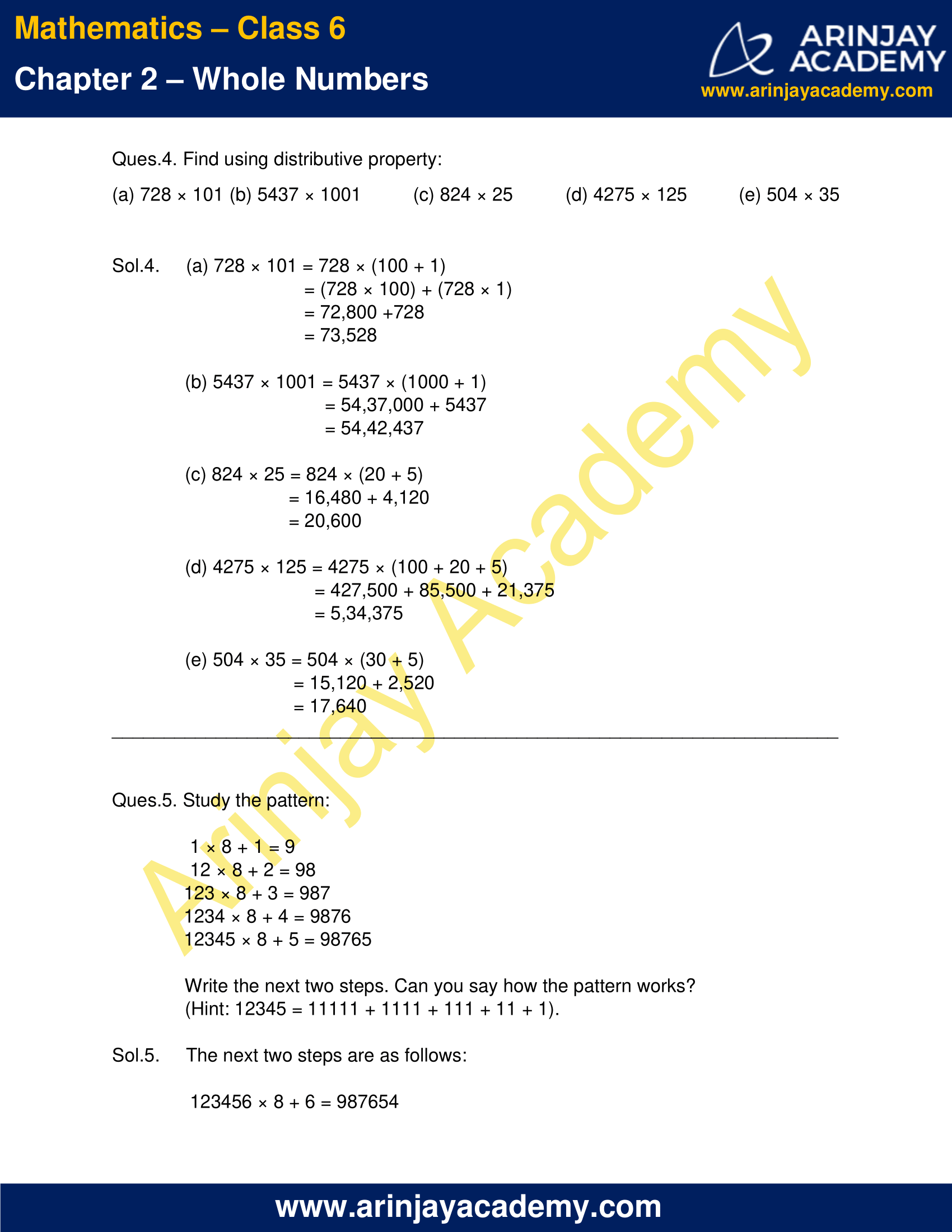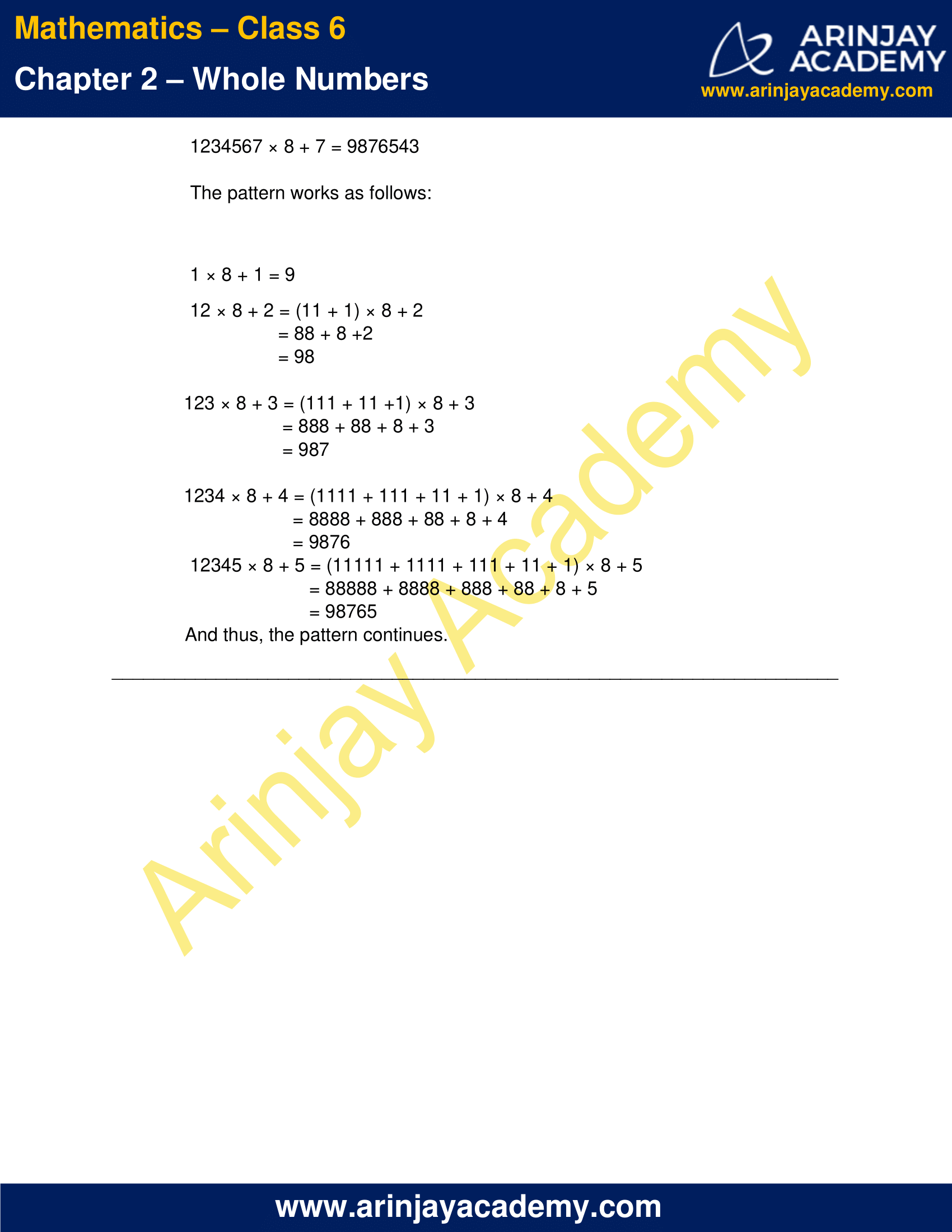### NCERT Solutions for Class 6 Maths Chapter 2 Exercise 2.1

Find the next natural numbers

Ques. 1. Write the next three natural numbers after 10999 ?

Solution 1.

The next number  after any given number, like 10999 in this case, can be found,  by adding 1 to the given number.

Now, the next three natural numbers after 10999, can be  as under : –

10999 + 1 = 11000 – (Adding 1 to 10999)
11000 + 1 = 11001 – (Adding 1 to 11000)
11001 + 1 = 11002 – (Adding 1 to 11001)

Three whole numbers occurring just before a given Number

Ques. 2. Write the three whole numbers occurring just before 10001.

Solution 2.

The three whole numbers occurring just before any given number , 10001 in the present case, can be  found by subtracting 1 from the number , then subtracting 1 from the result of first subtraction, and then subtracting 1 from the result of second subtraction :

First subtraction – 10001  1 = 10000 – ( Subtracting 1 from the number 10001 )
Second subtraction – 10000  1 = 9999 – ( Subtracting 1 from the number 10000,  the result of first subtraction)
Third subtraction – 9999  1 = 9998 – ( Subtracting 1 from the number 9999,  the result of second subtraction)

Finding the smallest whole number

Ques. 3. Which is the smallest whole number?

Solution 3.

Whole numbers start from 0 and  proceed as 0,1,2,3,4,5,6,7,8,9,10,…..

Hence, 0 (zero) is the smallest whole number.

Finding the whole numbers   between two given whole numbers

Ques. 4. How many whole numbers are there between 32 and 53?

Solution 4.

The whole numbers proceed at a difference of 1 from the previous number.

To find the number of whole numbers between any two numbers, we subtract the smaller number from the greater one, and subtract 1 from the answer.

Here, the number of whole numbers between 32 and 53 are found as follows:

53  32  1 = 20

Hence, there are 20 whole numbers between 32 and 53

Finding successor of a given number

Ques. 5. Write the successor of :

(a) 2440701
(b) 100199
(c) 1099999
(d) 2345670

Solution 5.

The successor of a number can be obtained    by adding 1  to the number. Accordingly the answer shall be as under :

(a) 2440701 + 1 = 2440702 – (Adding 1 to  2440701  )
(b) 100199 + 1 = 100200 – (Adding 1 to  100199)
(c) 1099999 +1 = 1100000 –  (Adding 1 to  1099999 )
(d) 2345670 + 1 = 2345671 – (Adding 1 to  2345670 )

Finding predecessor of a given number

Ques. 6. Write the predecessor of :

(a) 94
(b) 10000
(c) 208090
(d) 7654321

Solution 6.

The predecessor of a number can be obtained    by subtracting 1 from the number. Accordingly the answer shall be as under : –

(a) 94 – 1 = 93 – (Subtracting 1 from  94 )
(b) 10000 – 1 = 9999 – (Subtracting 1 from  10000 )
(c) 208090 – 1 = 208089 – (Subtracting 1 from 208090  )
(d) 208090 – 1 = 7654320 – (Subtracting 1 from  208090 )

Finding whole number which is on the left of the other number on the number line

Ques. 7. In each of the following pairs of numbers, state which whole number is on the left of the other number on the number line. Also write them with the appropriate sign (>, <) between them.

(a) 530, 503
(b) 370, 307
(c) 98765, 56789
(d) 9830415, 10023001

Solution 7.

On the number line containing whole numbers, the Smaller whole numbers shall lie on the left side of the larger numbers  .

(a) We know that 530 > 503

Hence, 503 shall lie on the left side of 530 on the number line.

(b) We know that 370 > 307

Hence, 307 shall lie   on the left side of 370 on the number line.

(c) We know that 98765 > 56789

Hence, 56789 shall lie on the left side of 98765 on the number line.

(d) We know that 10023001 > 9830415

Hence, 9830415 shall lie on the left side of 10023001 on the number line.

Ques. 8. Which of the following statements are true (T) and which are false (F) ?

(a) Zero is the smallest natural number.
(b) 400 is the predecessor of 399.
(c) Zero is the smallest whole number.
(d) 600 is the successor of 599.
(e) All natural numbers are whole numbers.
(f) All whole numbers are natural numbers.
(g) The predecessor of a two digit number is never a single digit number.
(h) 1 is the smallest whole number.
(i) The natural number 1 has no predecessor.
(j) The whole number 1 has no predecessor.
(k) The whole number 13 lies between 11 and 12.
(l) The whole number 0 has no predecessor.
(m) The successor of a two digit number is always a two digit number.

Solution:

(a) Statement – Zero is the smallest natural number.
Natural numbers start from 1 and proceed as 1,2,3,4,….
Hence, the above statement is false. The smallest natural number is 1 .

(b) Statement – 400 is the predecessor of 399.
Predecessor of a number is 1 less than the number.
So, (400-1 = 399) 399  is the predecessor of 400.
Hence, the above statement is false. 400 is the successor of 399.

(c) Statement – Zero is the smallest whole number.
Whole numbers start from 0 and proceed as 0,1,2,3,4,5….
Hence, the above statement is true.

(d) Statement – 600 is the successor of 599.
Successor of a number is one more than the number.
So, (599+1 = 600)  600 is the successor of 599.
Hence, the above statement is true.

(e) Statement – All natural numbers are whole numbers.
Natural numbers are all counting numbers starting from 1 as 1,2,3,4,….
Whole numbers are all natural numbers including 0.
Hence, the above statement is True.

(f) Statement – All whole numbers are natural numbers.
Zero is a whole number, but not a natural number.
Hence, the above statement is False.

(g) Statement – The predecessor of a two digit number is never a single digit number.
Predecessor of 10 (a two digit number) is 9 (a single digit number) .
Hence, the above statement is False.

(h) Statement – 1 is the smallest whole number.
Whole numbers start from 0. So, 0 is the smallest whole number.
Hence, the above statement is False.

(i) Statement – The natural number 1 has no predecessor.
Natural numbers start from 1. So, 1 is the smallest natural number.
Hence, the above statement is True.

(j) Statement – The whole number 1 has no predecessor.
Predecessor of number 1 is 0, which is a whole number.
Hence, the above statement is False.

(k) Statement – The whole number 13 lies between 11 and 12.
The above statement is False. since 13 lies after 12. There are no whole numbers between 11 and 12.

(l) Statement – The whole number 0 has no predecessor.
Whole numbers start from 0. So, 0 is the smallest whole number.
Hence, the above statement is True.

(m) Statement – The successor of a two digit number is always a two digit number.
The successor of 99 is 100, which is a 3 digit number.
Hence, the above statement is False.

### Class 6 Maths NCERT Solutions Chapter 2 – Exercise 2.2

Ques.1. Find the sum by suitable rearrangement:

(a) 837 + 208 + 363
(b) 1962 + 453 + 1538 + 647

Solution 1.

(a) 837 + 208 + 363 = 208 + (837 + 363)
( By Commutative & Associative property )
= 208 + 1200
= 1408

(b) 1962 + 453 + 1538 + 647 = (1962 + 1538) + (453 + 647)
= 3500 + 1100
= 4600

Ques.2. Find the product by suitable rearrangement:

(a) 2 × 1768 × 50
(b) 4 × 166 × 25
(c) 8 × 291 × 125
(d) 625 × 279 × 16
(e) 285 × 5 × 60
(f) 125 × 40 × 8 × 25

Solution 2.

(a) 2 × 1768 × 50 = 1768 × (2 × 50)
(By commutative and associative Property)
= 1,768 × 100
= 1,76,800

(b) 4 × 166 × 25 = 166 × (4 × 25)
(By commutative and associative Property)
= 166 × 100
= 16,600

(c) 8 × 291 × 125 = 291 × (8 × 125)
(By commutative and associative Property)
= 291 × 1000
= 2,91,000

(d) 625 × 279 × 16 = 279 × (625 × 16)
(By commutative and associative Property)
= 279 × 10,000
= 27,90,000

(e) 285 × 5 × 60 = 285 × (5 × 60)
(By commutative and associative Property)
= 285 × 300
= 85,500

(f) 125 × 40 × 8 × 25 = (125 × 8) × (40 × 25)
(By commutative and associative Property)
= 1000 × 1000
= 10,00,000

Ques.3. Find the value of the following:

(a) 297 × 17 + 297 × 3
(b) 54279 × 92 + 8 × 54279
(c) 81265 × 169 – 81265 × 69
(d) 3845 × 5 × 782 + 769 × 25 × 218

Solution 3.

(a) 297 × 17 + 297 × 3 = 297 × (17 + 3)
(By distributive property)
= 297 × 20
= 5,940

(b) 54279 × 92 + 8 × 54279 = 54279 × (92 + 8)
= 54279 × 100
= 54,27,900

(c) 81265 × 169 – 81265 × 69 = 81265 × (169 – 69)
= 81265 × 100
= 81,26,500

(d) 3845 × 5 × 782 + 769 × 25 × 218 = 3845 × 5 × 782 + (769 × 5) × 5 × 218
= 3845 × 5 × 782 + 3845 × 5 × 218
= 3845 × 5 × (782 + 218)
= 3845 × 5 × 1000
= 3845 × 5000
= 1,92,25,000

Ques.4. Find the product using suitable properties.

(a) 738 × 103
(b) 854 × 102
(c) 258 × 1008
(d) 1005 × 168

Solution 4.

(a) 738 × 103 = 738 × (100 + 3)
= (738 × 100) + (738 × 3)
= 73,800 + 2,214
= 76,014

(b) 854 × 102 = 854 × (100 + 2)
= (854 × 100) + (854 × 2)
= 85,400 + 1708
= 87,108

(c) 258 × 1008 = 258 × (1000 + 8)
= (258 × 1000) + (258 × 8)
= 258000 + 2064
= 2,60,064

(d) 1005 × 168 = (1000 + 5) × 168
= (1000 × 168) + (5 × 168)
= 168000 + 840
= 1,68,840

Ques.5. A taxidriver filled his car petrol tank with 40 litres of petrol on Monday. The next day, he filled the tank with 50 litres of petrol. If the petrol costs Rs 44 per litre, how much did he spend in all on petrol?

Solution 5.  Quantity of petrol filled by the taxi driver on Monday = 40 litres
Quantity of petrol filled by the taxi driver on the next day = 50 litres
Cost of petrol per litre = Rs 44
Total quantity of petrol filled by him = 40 + 50
= 90 litres
Total amount spent on petrol = Rs (44 × 90)
= Rs 3,960

Ques 6. A vendor supplies 32 litres of milk to a hotel in the morning and 68 litres of milk in the evening. If the milk costs Rs 15 per litre, how much money is due to the vendor per day?

Solution 6.  Quantity of milk supplied by the vendor in the morning = 32 litres
Quantity of milk supplied by the vendor in the evening = 68 litres
Total quantity of milk supplied by the vendor per day = (32+68) litres
= 100 litres
Cost of milk per litre = Rs 15
Amount due to the vendor per day = Rs (15 × 100)
= Rs 1500

Ques.7. Match the following: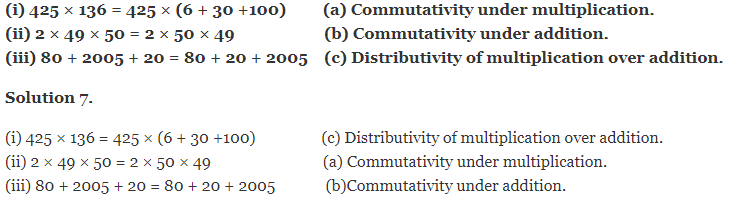### Class 6 Maths NCERT Solutions Chapter 2 – Exercise 2.3

Ques.1. Which of the following will not represent zero:

(a) 1 + 0
(b) 0 × 0
(c)  0/2
(d) (10-10)/2

Solution 1.

(a) 1 + 0 = 1

(b) 0 × 0 = 0

(c) 0/2= 0

(d) (10-10)/2 = 0

Hence, option (a) is the correct answer.

Ques.2. If the product of two whole numbers is zero, can we say that one or both of them will be zero? Justify through examples.

Solution 2. Yes, we can say that if the product of two whole numbers is zero, then either one or both of them are zeroes. This is because, any number multiplied by zero, becomes zero.

For example, 0 × 0 = 0
1 × 0 = 0
2 × 0 = 0… etc.

Ques.3. If the product of two whole numbers is 1, can we say that one or both of them will be 1? Justify through examples.

Solution 3.    If the product of two whole numbers is 1, then both of them should be 1. This is so, because 1 is the multiplicative identity for whole numbers. Hence, any number multiplied by 1, gives the number itself. In order to obtain the product of two whole numbers as 1, 1 should be multiplied by itself.

Ques.4. Find using distributive property:

(a) 728 × 101
(b) 5437 × 1001
(c) 824 × 25
(d) 4275 × 125
(e) 504 × 35

Solution 4.

(a) 728 × 101 = 728 × (100 + 1)
= (728 × 100) + (728 × 1)
= 72,800 +728
= 73,528

(b) 5437 × 1001 = 5437 × (1000 + 1)
= 54,37,000 + 5437
= 54,42,437

(c) 824 × 25 = 824 × (20 + 5)
= 16,480 + 4,120
= 20,600

(d) 4275 × 125 = 4275 × (100 + 20 + 5)
= 427,500 + 85,500 + 21,375
= 5,34,375

(e) 504 × 35 = 504 × (30 + 5)
= 15,120 + 2,520
= 17,640

Ques.5. Study the pattern:

1 × 8 + 1 = 9
12 × 8 + 2 = 98
123 × 8 + 3 = 987
1234 × 8 + 4 = 9876
12345 × 8 + 5 = 98765

Write the next two steps. Can you say how the pattern works?
(Hint: 12345 = 11111 + 1111 + 111 + 11 + 1).

Solution 5.

The next two steps are as follows:
123456 × 8 + 6 = 987654
1234567 × 8 + 7 = 9876543

The pattern works as follows:

1 × 8 + 1 = 9
12 × 8 + 2 = (11 + 1) × 8 + 2
= 88 + 8 +2
= 98

123 × 8 + 3 = (111 + 11 +1) × 8 + 3
= 888 + 88 + 8 + 3
= 987

1234 × 8 + 4 = (1111 + 111 + 11 + 1) × 8 + 4
= 8888 + 888 + 88 + 8 + 4
= 9876

12345 × 8 + 5 = (11111 + 1111 + 111 + 11 + 1) × 8 + 5
= 88888 + 8888 + 888 + 88 + 8 + 5
= 98765

And thus, the pattern continues.

With this we come to the end of NCERT Solutions for Class 6 Maths Chapter 2. We hope these helped you study your subject.